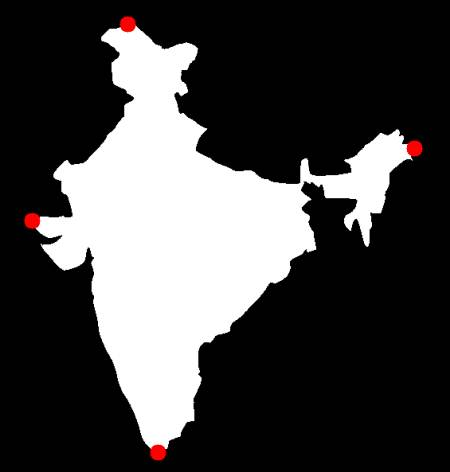# OpenCV-Python 系列 二十二 | 轮廓属性

### 目标

(注:质心、面积、周长等也属于这一类，但我们在上一章已经见过)

### 1. 长宽比

Aspect ; Ratio = frac{Width}{Height}

x,y,w,h = cv.boundingRect(cnt)
aspect_ratio = float(w)/h


### 2. 范围

Extent = frac{Object ; Area}{Bounding ; Rectangle ; Area}

area = cv.contourArea(cnt)
x,y,w,h = cv.boundingRect(cnt)
rect_area = w*h
extent = float(area)/rect_area


### 3. 坚实度

Solidity = frac{Contour ; Area}{Convex ; Hull ; Area}

area = cv.contourArea(cnt)
hull = cv.convexHull(cnt)
hull_area = cv.contourArea(hull)
solidity = float(area)/hull_area


### 4. 等效直径

Equivalent ; Diameter = sqrt{frac{4 times Contour ; Area}{pi}}

area = cv.contourArea(cnt)
equi_diameter = np.sqrt(4*area/np.pi)


### 5. 取向

(x,y),(MA,ma),angle = cv.fitEllipse(cnt)


### 6. 掩码和像素点

mask = np.zeros(imgray.shape,np.uint8)


### 7. 最大值，最小值和它们的位置

min_val, max_val, min_loc, max_loc = cv.minMaxLoc(imgray,mask = mask)


### 8. 平均颜色或平均强度

mean_val = cv.mean(im,mask = mask)


### 9. 极端点

leftmost = tuple(cnt[cnt[:,:,0].argmin()])
rightmost = tuple(cnt[cnt[:,:,0].argmax()])
topmost = tuple(cnt[cnt[:,:,1].argmin()])
bottommost = tuple(cnt[cnt[:,:,1].argmax()])### 练习

1. matlab的regionprops doc中仍然有一些特性。试着去实现它们。